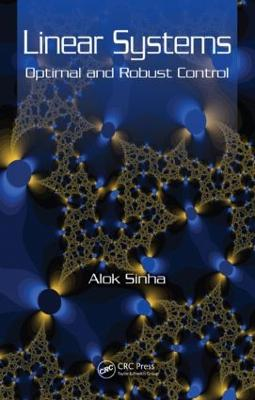•# Linear Systems: Optimal and Robust Control (Hardback)

(author)
£100.00
Hardback 488 Pages / Published: 31/01/2007
• We can order this

Usually dispatched within 3 weeks

Balancing rigorous theory with practical applications, Linear Systems: Optimal and Robust Control explains the concepts behind linear systems, optimal control, and robust control and illustrates these concepts with concrete examples and problems.

Developed as a two-course book, this self-contained text first discusses linear systems, including controllability, observability, and matrix fraction description. Within this framework, the author develops the ideas of state feedback control and observers. He then examines optimal control, stochastic optimal control, and the lack of robustness of linear quadratic Gaussian (LQG) control. The book subsequently presents robust control techniques and derives H control theory from the first principle, followed by a discussion of the sliding mode control of a linear system. In addition, it shows how a blend of sliding mode control and H methods can enhance the robustness of a linear system.

By learning the theories and algorithms as well as exploring the examples in Linear Systems: Optimal and Robust Control, students will be able to better understand and ultimately better manage engineering processes and systems.

Publisher: Taylor & Francis Inc
ISBN: 9780849392177
Number of pages: 488
Weight: 816 g
Dimensions: 229 x 152 x 31 mm